American Journal of Modern Physics
Volume 5, Issue 2-1, March 2016, Pages: 137-142

Study of Bose-Einstein Correlation Within the Framework of Hadronic Mechanics

Chandrakant S. Burande

Vilasrao Deshmukh College Engineering and Technology, Mouda, Nagpur, India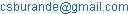Chandrakant S. Burande. Study of Bose-Einstein Correlation Within the Framework of Hadronic Mechanics. American Journal of Modern Physics. Special Issue: Issue II: Foundations of Hadronic Mechanics. Vol. 5, No. 2-1, 2016, pp. 137-142. doi: 10.11648/j.ajmp.2016050201.18

Received: July 8, 2015; Accepted: July 9, 2015; Published: June 1, 2016

Abstract: The Bose-Einstein correlation is the phenomenon in which protons and antiprotons collide at extremely high energies; coalesce one into the other resulting into the fireball of finite dimension. They annihilate each other and produces large number of mesons that remain correlated at distances very large compared to the size of the fireball. It was believed that special relativity and relativistic quantum mechanics are the valid frameworks to represent this phenomenon. Although, R.M. Santilli showed that the Bose-Einstein correlation requires four arbitrary parameters (chaoticity parameters) to fit the experimental data which parameters are prohibited by the basic axioms of relativistic quantum mechanics, such as that for the vacuum expectation values. Moreover, Santilli showed that correlated mesons can not be treated as a finite set of isolated point-like particles as required for the exact validity of the Lorentz and Poincare's symmetries, because the event is non-local due to overlapping of wavepackets and consequential non-Hamiltonian effects. Therefore, the Bose-Einstein correlation is incompatible with the axiom of expectation values of quantum mechanics. In this paper, we study Santilli's exact and invariant representation of the Bose-Einstein correlation via relativistic hadronic mechanics including the exact representation of experimental data from the first axiomatic principles without adulterations, and consequential exact validity of the Lorentz-Santilli and Poincare-Santilli isosymmetries under non-local and non-Hamiltonian internal effect. We finally study the confirmation of Santilli's representation of the Bose-Einstein correlation by F. Cardone and R. Mignani.
PACS: 13.38Dg, 03.30Tp, 10.30Cp, 02.20.Sv

Keywords: Bose-Einstein Correlation, Special Relativity, Lorentz-Santilli Isosymmetry

1. Introduction

The main ingredient of hadronic mechanics ,  is that strong interactions have a nonlocal component of contact, due to deep wave-overlappings at mutual distances of 1 Fermi. This nonlocal component can not be represented by the conventional quantum mechanics. However, novel hadronic mechanics encompass entire local and nonlocal effects with remarkable experimental evidences. Thus, the most fundamental experimental verifications of hadronic mechanics are, those which manifested the expected nonlocality of the strong interactions. Among them, the most important tests are those on the Bose-Einstein correlation , , , , , in which protons and antiprotons are made to collide at very big or very small energies and annihilate each other in a region called the fireball. The annihilation produces various unstable hadrons whose final states are given by correlated mesons which are "in phase" with each other despite large mutual distances compared to the size of the fireball. Correlated mesons can not be treated as a finite set of isolated point-like particles. It is non-local event due overlapping of wavepackets. There are several nonlocal theories which attempted to reduce nonlocal event into a finite set of isolated points distributed over the finite volume of the fireball. However, these theories are discarded by Santilli for the fact that the Bose-Einstein correlation is incompatible with the axiom of expectation values of quantum mechanics. It is purely manipulated nonlocal interaction to verify the quantum laws.

The first exact and invariant formulation of the Bose-Einstein correlation via relativistic hadronic mechanics was done by R. M. Santilli  in 1962. F. Cardone and R. Mignani ,  was the first to verify Santilli's theoretical isorelativistic calculation with experimental data (Figure 1) and they published their result in 1996.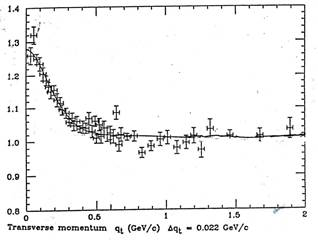Figure. 1. The exact fit of Santilli's two-point Bose-Einstein isocorrelation function at high energy.

2. Conventional Treatment of the Bose-Einstein Correlation

Consider a quantum system in 2-dimensions represented on a Hilbert space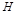with initial and final states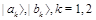. The vacuum expectation values of an operator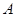are given by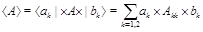(1)

which is necessarily diagonal, to fulfill the condition that operator corresponds to observable quantity must be Hermitian. The two-points correlation function of the Bose-Einstein correlation is defined by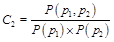(2)

where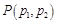is the two particles probability density subjected to Bose-Einstein symmetrization, and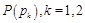is the corresponding quantity for theparticle with 4-momentum,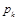. The two-particles density is computed via the vacuum expectation value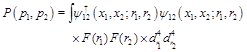(3)

where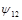is the probability amplitude to produce two bosons at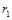and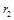that are detected at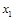and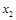, given by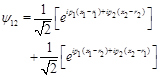(4)

With the use of above equations, we obtain the final expression for the two-point correlation function(5)

where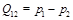is the momentum transfer, where R is the Gaussian width and r is generally assumed to be the radius of the fireball.

3. Incompatibility of the Bose-Einstein Correlation with Relativistic Quantum Mechanics

The Bose-Einstein correlation given by eq.(5) derived from conventional quantum mechanics, deviates from experimental results. This tempt to the introduction of a first, completely unknown parameter, called "chaoticity parameter", namely;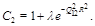(6)

Note that the introduction of chaoticity parameter is quite arbitrary and it is impossible to derive the above parameter from any axiom of relativistic quantum mechanics. Hence, the chaoticity parameterintroduced in eq.(6) is the first direct evidence of the incompatibility of the Bose-Einstein correlation with quantum axioms. Although, the modified eq.(6) too deviates dramatically from experimental data. In order to fit the desired experimental data eq.(6) was further modified by introducing an increasing number of completely unknown and arbitrary parameter, namely,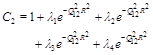(7)

which is strongly objected by Santilli because the only scientific route of achieving the addition terms in Bose-Einstein correlation function is by a formulation of nondiagonal elements of the expectation values. However, latter are prohibited by relativistic quantum mechanics for observable quantity (Hermitian operator). Thus, in the context of study of Bose-Einstein correlation, relativistic quantum mechanics has the following insufficiencies:

1. The theory can only represent the proton and the antiprotons as dimensionless points. Hence, the particle correlation and then the existence of the fireball is impossible with assumption of dimensionless points;

2. The point-like abstraction of particles has a number of technical consequences, such as the independent terms of the densities in eq.(3). That directly prohibit the boson correlation; and

3. Relativistic quantum mechanics must insist the fireball to be necessarily spherical, so as to prevent the loss of the rotational symmetry to protect from the violation of spacetime symmetries.

4. Representation of the Bose-Einstein Correlation with Relativistic Hadronic Mechanics

In regard of derivation of Bose-Einstein correlation function and its experimental validity, Santilli observed that:

1. The Bose-Einstein correlation is incompatible with the axisms of relativistic quantum mechanics because of the impossibility to admit off-diagonal terms in the two-point correlation function from unadulterated first principles, and other reasons; and

2. The Bose-Einstein correlation is directly compatible with the axioms of the covering relativistic hadronic mechanics because of the admission of nonlocal non-Hamiltonian interactions and the appearence of off-diagonal terms from first principles.

The axiom of isoexpectation value for relativistic hadronic mechanics  is given by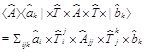(8)

where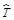is the isotopic element, and the "hat" denotes quantities defined on isospaces over isofields. The main new feature is that the operator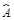must be Hermitian, thus diagonal, to be observable, but the isotopic element does not need to be diagonal. Santilli isorelativity with Minkowski-Santilli isospace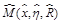, isoinvariant, isometric, isotopic element and isounit given respectively by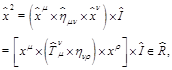(9)(10)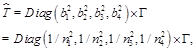(11)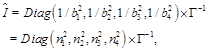(12)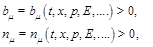(13)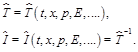(14)

It observed that unlike chaoticity parameter, the characteristic quantities must represent physically measurable quantities, namely,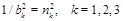must characterize the semiaxes of the Bose-Einstein fireball according to a proper normalization andmust characterize the density of the fireball in a way compatible with other experiments.

Note that the value of the type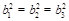emerging from isorepresentation would be inconsistant because the Bose-Ein stein fireball cannot possibly be a sphere due to the extreme energies of the collision. The fireball must be a very elongated sheroidalellipsoid, for instance, of the type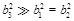. Moreover, the numerical value of the density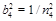must be compatible with numerical values from different experiments on comparable densities, such as we observed in case of protons and neutrons.

The correlation function on an iso-Hilbert space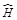with initial and final isostates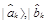;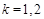and the non-diagonal isotopic element in the explicit form is given by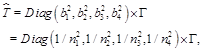(15)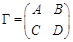(16)

where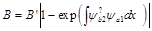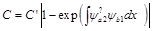and values of constants,and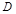are determined by the condition of normalization, that is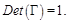(17)

Notice that isoexpectation value of eq.(11), isotopic element

1. Allows indeed off-diagonal terms in the isoexpectation values;

2. Represents the overlapping of the wavepackets of particles via the integrals in the exponents of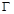; and

3. Eliminates all correlations when said overlapping is null.

Next, the isorepresentation is given by a trivial isotopy of the conventional treatment, with the use now of the nontrivial isoexpectation values of eq.(8). We then have the two-points isocorrelation function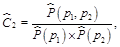(18)

where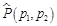is the two-particle isoprobability density subjected to proper symmetrization,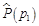and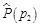are the corresponding quantity for the k particle with 4-momentum. The two-particles isoprobability density is now given by the isoeigenvalue expression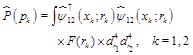(19)

Note that the crucial difference between eqs.(3) and (19) given by the isotopic lifting of all quantities and their operations and the appearance in the former of the isotopic element allowing the mixing of nondiagonal terms. Another major difference between conventional and isotopic treatments is that the probability densities for particles 1 and 2 are factorized in the conventional treatment, eq.(3), while they cannot be factorized in the isotopic treatment. This is due to the fact that protons, antiprotons, and all produced mesons are pointlike for relativistic quantum mechanics, while they are extended in case Santilli's treatment. Hence, the separation of the densities would be equivalent to ceasing all correlations. The isotopy of of the conventional treatment referred to isoexpectation values of eq.(11), including the symmetrization of the isotopic element and isowavefunctions for all possible directions, plus the assumed normalizations then leads to isodensity, that is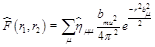(20)

where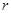is the radius of the sphere in which the correlated mesons are detected.

The continuation of calculations via a simple isotopy of the conventional treatment, the final expression of the two-points isocorrelation function, derived for the first time by Santilli is given by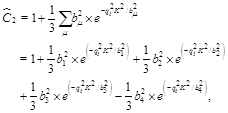(21)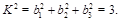(22)

In the above isorepresentations, all operations are now conventional. Hence, the above expressions are the projections in our spacetime of the isocorrelation functions on isospace.

5. Exact Poincare Symmetry under Nonlocal and Non-Hamiltonian Interaction

As indicated earlier, a crucial insufficiency of the conventional treatment of the Bose-Einstein correlation, is the inability to provide an invariant representation of the fireball, due to its prolate character under which the conventional rotational symmetry no longer applies. The Bose-Einstein correlation creates a fireball characterized by a spheroid prolated in the direction of the proton-antiproton flight. Following its creation, the fireball expands rapidly, resulting in the correlated mesons. Consequently, the original characteristic quantities, here denoted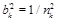, have an explicit dependence on time. By assuming that the prolateness is along the third axis, we have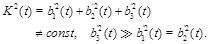(23)

However, the fireball must preserve its shape during its expansion when considered as isolated from the rest of the universe. This implies that all characteristic quantities have the same factorizable time dependence. In conclusion, the fireball can be studied at the time of its formation with constant characteristic quantities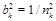and the following isoinvariant formulated on the Euclide-Santilli isospace ,  with isounit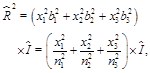(24)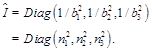(25)

The reconstruction of the exact Lorentz symmetry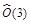,  for the Bose-Einstein correlation follows the same lines. Since the speed of light is assumed to be locally varying, we have mutated light cones of the type,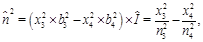(26)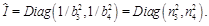(27)

It is again easy to see that the mutated light cone in our spacetime is the perfect light cone in isospace, called light isocone, because, again, the mutation of each axis is complemented by the inverse mutation of the corresponding unit. Recall that isorelativity and special relativity coincide at the abstract, realization free level, as confirmed by the speed of light in vacuum to be the constant maximal causal speed in isospace. Consequently, the understanding of the isorepresentation of the Bose-Einstein correlation requires the knowledge that, rather than "violating" special relativity as at times perceived, in reality allows the maximal possible enlargement of the arena of applicability of Einsteinian axioms.

6. Theoretical Prediction

It is important now to identify the theoretical prediction of isorepresentation so that we can compared them below with experimental data.

Prediction 1: The minimum value of the two-points isocorrelation function, first identified by Santilli, evidently holding for infinite momentum transfer.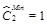(28)

Prediction 2: The maximal value is predicted to be evidently holding for null momentum transfer. The isorepresentation to be valid if all data must remain between the above minimum and maximum values.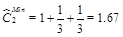(29)

Prediction 3: Isorepresentation also predicts the maximum value of the isodensity, occurring for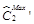. In fact, for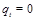we have no correlations, in which case we have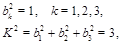(30)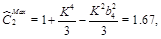(31)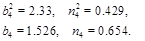(32)

Prediction 4: By assuming that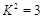and that the fireball is very prolate,with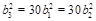, we obtain the following prediction on the remaining characteristic quantities From the isoaxioms, Santilli also have the following additional predictions: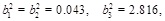(33)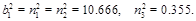(34)

Prediction 5: The maximal causal speed within the fireball is bigger than that in vacuum,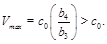(35)

Prediction 6: Time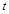within the fireball flows faster than time predicted by special relativity,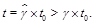(36)

Prediction 7: Lengths 'inside the fireball are smaller than lengths predicted by special relativity,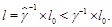(37)

Prediction 8: Mass behavior with speed is bigger than that predicted by special relativity,(38)

Prediction 9: The energy equivalence of the fireball is bigger than that predicted by special relativity or, equivalently, for a given energy, the mass is smaller,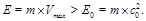(39)

Prediction 10 : Frequencies of light emitted inside the fireball, exist the same isoblueshifted, namely, with an increase of frequency as compared to the corresponding behavior predicted by special relativity,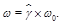(40)

Prediction 11: The speed of light within the fireball is bigger than that in vacuum,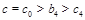, by smaller than the maximal causal speed,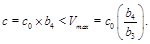(41)

The isoblueshift of light is nothing mysterious because it is a mere manifestation of the high energy density of the medium in which light propagates. Isoblueshift, as the increase of frequencies as predicted by special relativity in vacuum, is then a mere consequence of the medium transfer energy to light. A similar situation occurs for all other predictions.

7. Experimental Verification

F. Cardone and R. Mignani ,  in 1992 had contested the eq.(15) for actual experimental data. The Bose-Einstein two-point correlation function derived by Santilli is perfectly matched with experimental results at high energy. The numerical values of the characteristic functions for the fireball of the Bose-Einstein correlation resulting from this exercise are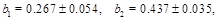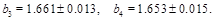(42)

A most important feature of the above data is that they characterize the medium inside the fireball as being iso-Minkowskian of Group III, Type 9, thus confirming that all hadrons heavier than kaons have the same iso-Minkowskian features. The fit of FIGURE 1 and the above values provide the following experimental verifications:

1. The experimental data do indeed lie between the theoretically minimum and maximal value;

2.  The experimental data confirm all eleven theoretical predictions;

3.The experimental proof confirms the reconstruction of the exact character of the Poincare symmetry for the Bose-Einstein correlation.

F. Cardone and R. Mignani investigation provides remarkable experimental verification of Santilli isorelativity and relativistic hadronic mechanics. This experimental verification on Bose-Einstein Correlation reveals the nonlocality of strong interactions of correlated mesons.

8. Concluding Remarks

Santilli's thorough investigation found that special relativity, the Lorentz and Poincare's symmetries, and relativistic quantum mechanics are not exactly valid to represent the Bose-Einstein correlation because their predictions from a large deviation from experimental data. Moreover, the resulting representations fail to tender non-explanation for the introduction of chaoticity parameters which are needed to fit the experimental data. Furthermore, it is observed that there are flaws in treating correlated mesons as a finite set of isolated point-like particles, sincethe Bose-Einstein correlation is purely non-local event with deep overlapping of wavepackets that cannot be treated by conventional quantum mechanics.

We have shown in this paper that Santilli's representation of the Bose-Einstein correlation via relativistic hadronic mechanics has indeed achieved a numerically exact and and time invariant representation of experimental data from unadulterated first axioms, with consequential exact validity of the Lorentz-Santilli and Poincare-Santilli isosymmetry that are known to be locally isomorphic to the corresponding conventional symmetry. Hence, relativistic hadronic mechanics restores indeed the validity of the conventional spacetime symmetries, although at the covering isotopic level. We conclude the paper with a study of the confirmation of all results by Santilli independently provided by F. Cardone and R. Mignani, including the all important exact and invariant representation experimental data.

Acknowledgments

The author is highly grateful to Professor R. M. Santilli, his team and Professor Anderson for valuable guidance in preparing and for suggestions to improve this paper. The author is also highly indebted to Professor A. A. Bhalekar for suggesting me this topic and helping me in writing this paper by his valuable advices and suggestions in its overall improvement.

References

1. R. M. Santilli, Hadronic Mathematics, Mechanics and Chemistry, Vol. I-V, International Academic Press, Palm Harbor, U.S.A., 2008.
2. I. Gandzha and J. Kadeisvily, New Sciences for a New Era: Mathematical, Physical and Chemical Discoveries of Ruggero Maria Santilli, The Institute for Basic Research, Palm Harbor, Florida, U.S.A., 2011.
3. B. Lorstad, Int. J. Mod. Phys. A 4, 2861 (1989).
4. D. H. Boal et al. Rev. Mod. Phys. 62, 553 (1990).
5. R. Adler et al., Z. Phys. C 63, 541 (1994).
6. M. Gaspero et al., Phys. Lett. B 58, 861 (1995).
7. G. Goldhaber, S. Goldhaber, W. Lee, and A. Pais, Phys. Rev. 120, 300, (1960).
8. R. M. Santilli, Hadronic J. 15, 1 and 81 (1992).
9. F. Cardone and R. Mignani, JETP, 83, 435  (1996).
10. F. Cardone, M. Gasperini and R. Mignani Euop.       Phys. J. C 4, 705 (1998).
11. R. M. Santilli,Lie-admissible Approach to the Hadronic Structure, Volume I: Non-applicability of the Galilei and Einstein Relativities in the series Monographs in Theoretical Physics, Hadronic Press, Palm Harbor, Florida, 1978.
12. R. M. Santilli, Lie-admissible Approach to the Hadronic Structure, Volume II: Coverings of the Galilei and Einstein Relativities in the series Monographs in Theoretical Physics, Hadronic Press, Palm Harbor, Florida, 1981.

 Contents 1. 2. 3. 4. 5. 6. 7. 8.
Article ToolsAbstractPDF(340K)